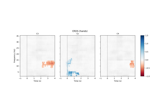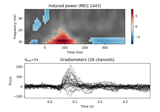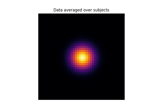# mne.stats.permutation_cluster_1samp_test¶

mne.stats.permutation_cluster_1samp_test(X, threshold=None, n_permutations=1024, tail=0, stat_fun=None, adjacency=None, n_jobs=1, seed=None, max_step=1, exclude=None, step_down_p=0, t_power=1, out_type=None, check_disjoint=False, buffer_size=1000, connectivity=None, verbose=None)[source]

Non-parametric cluster-level paired t-test.

Parameters
Xarray, shape (n_observations, p[, q])

The data to be clustered. The first dimension should correspond to the difference between paired samples (observations) in two conditions. The subarrays X[k] can be 1D (e.g., time series) or 2D (e.g., time-frequency image) associated with the kth observation. For spatiotemporal data, see also mne.stats.spatio_temporal_cluster_1samp_test().

threshold

If numeric, vertices with data values more extreme than threshold will be used to form clusters. If threshold is None, a t-threshold will be chosen automatically that corresponds to a p-value of 0.05 for the given number of observations (only valid when using a t-statistic). If threshold is a dict (with keys 'start' and 'step') then threshold-free cluster enhancement (TFCE) will be used (see the TFCE example and 1).

n_permutationsint | ‘all’

The number of permutations to compute. Can be ‘all’ to perform an exact test.

tailint

If tail is 1, the statistic is thresholded above threshold. If tail is -1, the statistic is thresholded below threshold. If tail is 0, the statistic is thresholded on both sides of the distribution.

stat_fun

Function called to calculate the test statistic. Must accept 1D-array as input and return a 1D array. If None (the default), uses mne.stats.ttest_1samp_no_p().

Defines adjacency between locations in the data, where “locations” can be spatial vertices, frequency bins, etc. If False, assumes no adjacency (each location is treated as independent and unconnected). If None, a regular lattice adjacency is assumed, connecting each location to its neighbor(s) along the last dimension of X (or the last two dimensions if X is 2D). If adjacency is a matrix, it is assumed to be symmetric (only the upper triangular half is used) and must be square with dimension equal to X.shape[-1] or X.shape[-1] * X.shape[-2] .

n_jobsint

The number of jobs to run in parallel (default 1). Requires the joblib package.

seedNone | int | instance of RandomState

If seed is an int, it will be used as a seed for RandomState. If None, the seed will be obtained from the operating system (see RandomState for details). Default is None.

max_stepint

Maximum distance along the second dimension (typically this is the “time” axis) between samples that are considered “connected”. Only used when connectivity has shape (n_vertices, n_vertices).

exclude

Mask to apply to the data to exclude certain points from clustering (e.g., medial wall vertices). Should be the same shape as X. If None, no points are excluded.

step_down_pfloat

To perform a step-down-in-jumps test, pass a p-value for clusters to exclude from each successive iteration. Default is zero, perform no step-down test (since no clusters will be smaller than this value). Setting this to a reasonable value, e.g. 0.05, can increase sensitivity but costs computation time.

t_powerfloat

Power to raise the statistical values (usually t-values) by before summing (sign will be retained). Note that t_power=0 will give a count of locations in each cluster, t_power=1 will weight each location by its statistical score.

Output format of clusters. If 'mask', returns boolean arrays the same shape as the input data, with True values indicating locations that are part of a cluster. If 'indices', returns a list of lists, where each sublist contains the indices of locations that together form a cluster. Note that for large datasets, 'indices' may use far less memory than 'mask'. The default translates to 'mask' in version 0.21 but will change to 'indices' in version 0.22.

check_disjointbool

Whether to check if the connectivity matrix can be separated into disjoint sets before clustering. This may lead to faster clustering, especially if the second dimension of X (usually the “time” dimension) is large.

buffer_size

Block size to use when computing test statistics. This can significantly reduce memory usage when n_jobs > 1 and memory sharing between processes is enabled (see mne.set_cache_dir()), because X will be shared between processes and each process only needs to allocate space for a small block of locations at a time.

connectivityNone

Deprecated and will be removed in 0.22, use adjacency instead.

verbose

If not None, override default verbose level (see mne.verbose() and Logging documentation for more). If used, it should be passed as a keyword-argument only.

Returns
t_obsarray, shape (n_tests,)

T-statistic observed for all variables.

clusterslist

List type defined by out_type above.

cluster_pvarray

P-value for each cluster.

H0array, shape (n_permutations,)

Max cluster level stats observed under permutation.

Notes

From an array of paired observations, e.g. a difference in signal amplitudes or power spectra in two conditions, calculate if the data distributions in the two conditions are significantly different. The procedure uses a cluster analysis with permutation test for calculating corrected p-values. Randomized data are generated with random sign flips. See 2 for more information.

Because a 1-sample t-test on the difference in observations is mathematically equivalent to a paired t-test, internally this function computes a 1-sample t-test (by default) and uses sign flipping (always) to perform permutations. This might not be suitable for the case where there is truly a single observation under test; see Statistical inference.

If n_permutations >= 2 ** (n_samples - (tail == 0)), n_permutations and seed will be ignored since an exact test (full permutation test) will be performed.

If no initial clusters are found, i.e., all points in the true distribution are below the threshold, then clusters, cluster_pv, and H0 will all be empty arrays.

References

1

Stephen M. Smith and Thomas E. Nichols. Threshold-free cluster enhancement: addressing problems of smoothing, threshold dependence and localisation in cluster inference. NeuroImage, 44(1):83–98, 2009. doi:10.1016/j.neuroimage.2008.03.061.

2

Eric Maris and Robert Oostenveld. Nonparametric statistical testing of EEG- and MEG-data. Journal of Neuroscience Methods, 164(1):177–190, 2007. doi:10.1016/j.jneumeth.2007.03.024.

## Examples using mne.stats.permutation_cluster_1samp_test¶